# Triangular tiling

Triangular tiling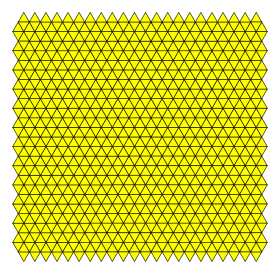TypeRegular tiling
Vertex configuration3.3.3.3.3.3 (or 36)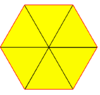Schläfli symbol(s){3,6}
{3}
Wythoff symbol(s)6 | 3 2
3 | 3 3
| 3 3 3
Coxeter diagram(s)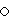=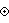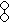Symmetryp6m, [6,3], (*632)
Rotation symmetryp6, [6,3]+, (632)
p3, [3]+, (333)
DualHexagonal tiling
PropertiesVertex-transitive, edge-transitive, face-transitive

In geometry, the triangular tiling or triangular tessellation is one of the three regular tilings of the Euclidean plane. Because the internal angle of the equilateral triangle is 60 degrees, six triangles at a point occupy a full 360 degrees. The triangular tiling has Schläfli symbol of {3,6}.

Conway calls it a deltille, named from the triangular shape of the Greek letter delta (Δ). The triangular tiling can also be called a kishextille by a kis operation that adds a center point and triangles to replace the faces of a hextille.

It is one of three regular tilings of the plane. The other two are the square tiling and the hexagonal tiling.

## Uniform colorings

There are 9 distinct uniform colorings of a triangular tiling. (Naming the colors by indices on the 6 triangles around a vertex: 111111, 111112, 111212, 111213, 111222, 112122, 121212, 121213, 121314) Three of them can be derived from others by repeating colors: 111212 and 111112 from 121213 by combining 1 and 3, while 111213 is reduced from 121314.

There is one class of Archimedean colorings, 111112, (marked with a *) which is not 1-uniform, containing alternate rows of triangles where every third is colored. The example shown is 2-uniform, but there are infinitely many such Archimedean colorings that can be created by arbitrary horizontal shifts of the rows.

 111111 121212 111222 112122 111112(*)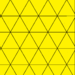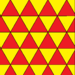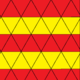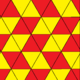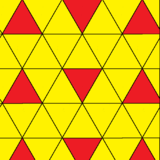p6m (*632) p3m1 (*333) cmm (2*22) p2 (2222) p2 (2222)
 121213 111212 111112 121314 111213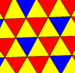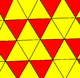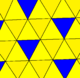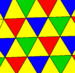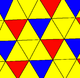p31m (3*3) p3 (333)

## A2 lattice and circle packings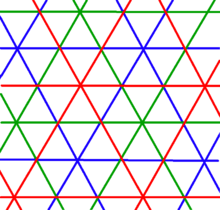The A*
2
lattice as three triangular tilings:+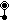+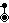The vertex arrangement of the triangular tiling is called an A2 lattice. It is the 2-dimensional case of a simplectic honeycomb.

The A*
2
lattice (also called A3
2
) can be constructed by the union of all three A2 lattices, and equivalent to the A2 lattice.++= dual of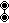=The vertices of the triangular tiling are the centers of the densest possible circle packing. Every circle is in contact with 6 other circles in the packing (kissing number). The packing density is π12 or 90.69%. Since the union of 3 A2 lattices is also an A2 lattice, the circle packing can be given with 3 colors of circles.

The voronoi cell of a triangular tiling is a hexagon, and so the voronoi tessellation, the hexagonal tiling has a direct correspondence to the circle packings.

A2 lattice circle packing A*
2
lattice circle packing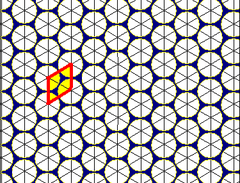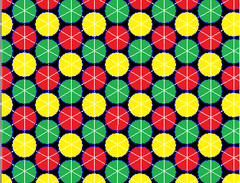## Geometric variations

Triangular tilings can be made with the equivalent {3,6} topology as the regular tiling (6 triangles around every vertex). With identical faces (face-transitivity) and vertex-transitivity, there are 5 variations. Symmetry given assumes all faces are the same color.

The planar tilings are related to polyhedra. Putting fewer triangles on a vertex leaves a gap and allows it to be folded into a pyramid. These can be expanded to Platonic solids: five, four and three triangles on a vertex define an icosahedron, octahedron, and tetrahedron respectively.

This tiling is topologically related as a part of sequence of regular polyhedra with Schläfli symbols {3,n}, continuing into the hyperbolic plane.

It is also topologically related as a part of sequence of Catalan solids with face configuration Vn.6.6, and also continuing into the hyperbolic plane.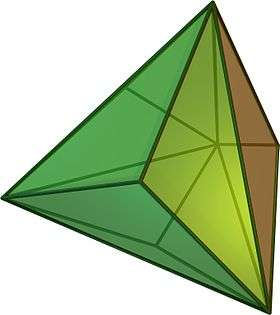V3.6.6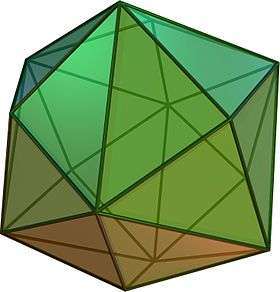V4.6.6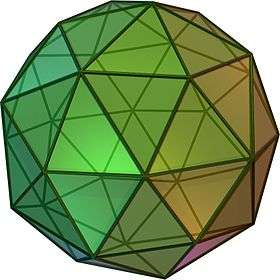V5.6.6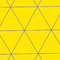V6.6.6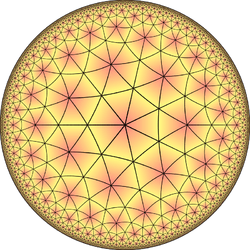V7.6.6

### Wythoff constructions from hexagonal and triangular tilings

Like the uniform polyhedra there are eight uniform tilings that can be based from the regular hexagonal tiling (or the dual triangular tiling).

Drawing the tiles colored as red on the original faces, yellow at the original vertices, and blue along the original edges, there are 8 forms, 7 which are topologically distinct. (The truncated triangular tiling is topologically identical to the hexagonal tiling.)

There are 4 regular complex apeirogons, sharing the vertices of the triangular tiling. Regular complex apeirogons have vertices and edges, where edges can contain 2 or more vertices. Regular apeirogons p{q}r are constrained by: 1/p + 2/q + 1/r = 1. Edges have p vertices, and vertex figures are r-gonal.

The first is made of 2-edges, and next two are triangular edges, and the last has overlapping hexagonal edges.

2{6}6 or3{4}6 or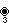3{6}3 or6{3}6 or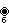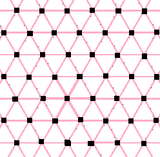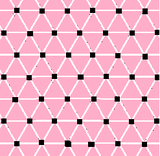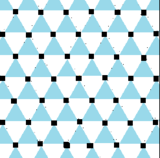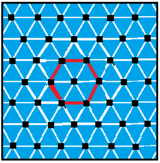### Other triangular tilings

There are also three Laves tilings made of single type of triangles: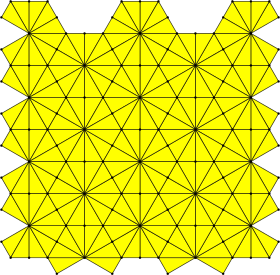Kisrhombille30°-60°-90° right triangles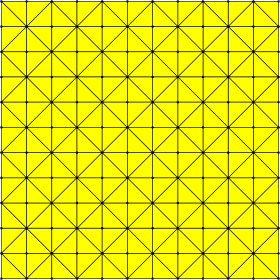Kisquadrille45°-45°-90° right triangles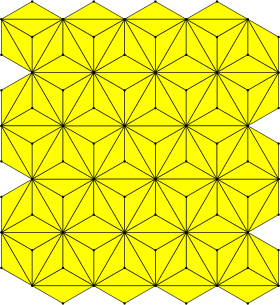Kisdeltile30°-30°-120° isosceles trianglesWikimedia Commons has media related to Order-6 triangular tiling.
1. Tilings and Patterns, p.102-107
2. http://www.math.rwth-aachen.de/~Gabriele.Nebe/LATTICES/A2.html
3. Order in Space: A design source book, Keith Critchlow, p.74-75, pattern 1
4. Tilings and Patterns, from list of 107 isohedral tilings, p.473-481
5. Coxeter, Regular Complex Polytopes, pp. 111-112, p. 136.
• Coxeter, H.S.M. Regular Polytopes, (3rd edition, 1973), Dover edition, ISBN 0-486-61480-8 p. 296, Table II: Regular honeycombs
• Grünbaum, Branko ; and Shephard, G. C. (1987). Tilings and Patterns. New York: W. H. Freeman. ISBN 0-7167-1193-1. (Chapter 2.1: Regular and uniform tilings, p. 58-65, Chapter 2.9 Archimedean and Uniform colorings pp.102-107)
• Williams, Robert (1979). The Geometrical Foundation of Natural Structure: A Source Book of Design. Dover Publications, Inc. ISBN 0-486-23729-X. p35
• John H. Conway, Heidi Burgiel, Chaim Goodman-Strass, The Symmetries of Things 2008, ISBN 978-1-56881-220-5

Fundamental convex regular and uniform honeycombs in dimensions 3–10 (or 2-9)
Family//Uniform tiling {3} δ3 hδ3 qδ3 Hexagonal
Uniform convex honeycomb {3} δ4 hδ4 qδ4
Uniform 5-honeycomb {3} δ5 hδ5 qδ5 24-cell honeycomb
Uniform 6-honeycomb {3} δ6 hδ6 qδ6
Uniform 7-honeycomb {3} δ7 hδ7 qδ7 222
Uniform 8-honeycomb {3} δ8 hδ8 qδ8 133331
Uniform 9-honeycomb {3} δ9 hδ9 qδ9 152251521
Uniform 10-honeycomb {3} δ10 hδ10 qδ10
Uniform n-honeycomb {3[n]} δn hδn qδn 1k22k1k21